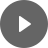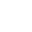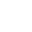18. Networks - StudyNinja
Select Page

HSC Standard Maths Resources

Browse: 1. Home  » 18. Networks

18. Networks

Get access to 20 Mock Exams with over 700 exam-style questions for HSC Standard Maths.

This is one of the two new topics that have been added to the latest syllabus. In 2020 exams three questions appeared from this topic worth 5 marks.

Basics

When it comes to networks, the following are a few basic things you need to understand.Example 1

For the network diagram below, answer the following questions:1. Make a table showing vertex and number of degrees of each vertex
2. What is the total number of edges?
3. How many loops are there?

Solution 1

1.2. Total number of edges is total number of blue lines which is 5.

3. There is only one loop at vertex D.

Example 2

Draw the network diagram from the following table and answer the following questions:What is the indegree and outdegree at A and E?

Solution 2

Note there are many ways of drawing the network; the important thing is that the information in the table is conveyed correctly.There are two edges pointing out form vertex A and one edge pointing inwards, so the outdegree at A is 2 and indegree is 1, while the outdegree at E is 0 and indegree is 4.

Walk, path and cycle

Consider the following network:Walk is any combination of vertices and edges. So ACDB is a walk, ABDEDB is a walk, ACDEB is a walk etc. However, AE is not a walk as there is no edge between them.

Path is a special type of walk that doesn’t visit any vertex more than once. ABDC is a path but ABDCA is not a path.

Cycle, as the name suggests, is a walk that completes cycle by joining the starting and ending vertex and doesn’t touch any other vertex more than once. ABDCA is a cycle, BDEB is a cycle.

Tree

In the network above, there is more than one way of connecting some of the vertices. E.g. A and E can be connected via ABE or ABDE or ACDE.

This kind of a network is not a tree. A tree is a network in which there is only one way of connecting any two vertices together. For example, the following network is a tree.Example 3

Consider the following network:1. Determine a path from A to D.
2. Identify one cycle from vertex B.
3. Is this network a tree? Why? If not, suggest one change that will make it a tree.

Solution 3

1. One path can be ABCD
2. BCDEB (Starts and finishes at B and does not touch any vertex other than B more than once)
3. This network is not a tree as there is more than one way of connecting some vertices. E.g. ABCD and ABED are two ways of connecting A with D. One of the ways of making it a tree can be as follows; if connection between E and B is disconnected, the network will become a tree.

Algorithms

There are times when you have to figure out the most ‘efficient’ network where definition of ‘efficient’ varies from problem to problem.

Imagine you have the following network showing distance between different points in a house (Assume connection between B and C is practically not possible):A problem on hand can be to find the most efficient path for wiring that connects all vertices and uses the minimum distance to minimize costs.

Before coming to the algorithms that solve this kind of problems, we first define spanning tree. Spanning tree contains all the vertices of the network and the minimum number of edges that connects all those vertices (so there aren’t any cycles).

Also, remember that the number of edges in a spanning tree will always be total vertices – 1.

The reason for considering spanning trees is that in situations like above, it is inefficient to do wiring using all possible connections; instead, it should be done using the most cost-efficient manner and to determine that first step is to determine different ways in which connections can be made (or different spanning trees), and then the question comes around which one minimizes it.

The following are all different spanning trees from the network above:From all the spanning trees above, it is obvious that the connecting CABD is the most efficient as the sum of the weights is the minimum there. This tree is called the minimum spanning tree.

It is very time-consuming to draw all the possible combinations of a network and to work out the minimum spanning tree. This is where algorithms help. We will be considering two algorithms for the minimum spanning problems:

• Prim’s algorithm
• Kruskal’s algorithm

Example 4

Consider the following network:Determine the minimum spanning tree using i) Prim’s algorithm ii) Kruskal’s algorithm.

Solution 4

Using Prim’s algorithm:Start from any vertex. Starting from A here.

Consider all possible edges from A.

Select the shortest. AC in this case.Now include vertex C in consideration.

Repeat the same process.

AB to be selected.Repeat the same process.

BD selected at this stage.Because CD will complete a cycle, it is not considered.

BE selected at this stage.DE removed from consideration.

AF selected.Final tree with CF removed from consideration

Using Kruskal’s algorithm:Select edge with the minimum weight.

BD selected.Continue the same process while keeping an eye on any cycles.

BE selected.DE removed.

AC selected.AB selected.AF selected.

CF removed.The final minimum spanning tree.

There are times when connecting to each vertex is not important like going from one point to another and all you care is the shortest distance to reach between two points.

In this kind of situations, Dijkstra’s algorithm is helpful to determine the shortest path.

Example 5

Consider the following network. Find the shortest distance from A to H.Solution 5

Dijkstra’s algorithm is quite similar to Prim’s algorithm where you start from a vertex (in Prim’s case it can be any vertex, which is not the case with Dijkstra).

In Prim’s algorithm, you consider and select the next smallest weight edge as you go through circuit without worrying about the total weight, where in Dijkstra’s algorithm you will do the same but you will also consider the total weight as you go along vertices.Start from vertex A as question asks about shortest path from vertex A.

AF is the shortest among all options so select it.AFG has a total weight of 5 which is higher than AB’s weight of 4, so AB is selected.Continuing on AFG has the least weight.AFGE has the least weight now.ABC has the least weight now.ABCD, AFGEH and AE have the same weight now, but AFGEH leads to the destination in the shortest possible way.Therefore, the shortest distance is AFGEH.

You might find the following videos helpful related to this section:2A Network Terminology (1 of 2)

by Mr Bodgers (click to view channel)

An introductory video to network diagrams.2A Network Terminology (2 of 2)

by Mr Bodgers (click to view channel)

Explains the terminology used when working with network diagrams.2B Travelling a Network (1 of 3)

by Mr Bodgers (click to view channel)

Explains the terminology used when travelling a network.2B Travelling a Network (2 of 3)

by Mr Bodgers (click to view channel)

Completes an example where you describe the route taken on a network diagram.2B Travelling a Network (3 of 3)

by Mr Bodgers (click to view channel)

xplains of a traversable and non-traversable graph.2C Drawing Network Diagrams (1 of 6)

by Mr Bodgers (click to view channel)

Explains what connected graphs are.2C Drawing Network Diagrams (2 of 6)

by Mr Bodgers (click to view channel)

Explains what an isomorphic graph is.2C Drawing Network Diagrams (3 of 6)

by Mr Bodgers (click to view channel)

Completes an example where you draw isomorphic graphs.2C Drawing Network Diagrams (4 of 6)

by Mr Bodgers (click to view channel)

Explains how to represent a map as a network diagram.2C Drawing Network Diagrams (5 of 6)

by Mr Bodgers (click to view channel)

Completes an example where you represent a table as a network diagram.2C Drawing Network Diagrams (6 of 6)

by Mr Bodgers (click to view channel)

Completes an example where you convert a weight network diagram to a table.The following is the type of questions you can expect in exam:

Study notes of this section and other resources can be accessed here:

Browse: 1. Home  » 18. Networks